/* styles */ Hi DC Community!

# The Desert Cove eNews is sent on a MONTHLY basis.

If your family has moved on from Desert Cove, or you do not wish to receive the Desert Cove PTO eNews, please click 'Unsubscribe' at the bottom of this emailSEPTEMBER 2019

NOTE: Any links below will take you to more details!

▪ Sep 6: DC Family Bingo Night
▪ Sep 7: SMHS Matadors in Training Cheer Clinic (Register)
▪ Sep 10-13: Health Screenings (VOLUNTEERS NEEDED)
▪ Sep 13: SMHS Matadors in Training Half-Time Performance
▪ Sep 17: DC Spirit Night @ Habit Burger
▪ Sep 18: United Parent Council Meeting
▪ Sep 24: PTO Meeting @ 6PM in Library
▪ Sep 25-27: Fall Book Fair (VOLUNTEERS NEEDED)
▪ Sep 30: NO SCHOOL
 ▪ Sep 6: DC Family Bingo Night
 ▪ Sep 10-13: Health Screenings (VOLUNTEERS NEEDED)
 ▪ Sep 17: DC Spirit Night @ Habit Burger
 ▪ Sep 18: United Parent Council Meeting
 ▪ Sep 24: PTO Meeting @ 6PM in Library
 ▪ Sep 25-27: Fall Book Fair (VOLUNTEERS NEEDED)
 ▪ Sep 30: NO SCHOOL

 table div table+table+table+table+table+table div table{width:100%;padding:0}table div table+table+table+table+table+table div table img{width:96.23%;padding:0;float:none}table div table+table+table+table+table+table div table td{width:100%;padding:0 1.88% 18px}/* styles */## Family Bingo Night is TONIGHT!!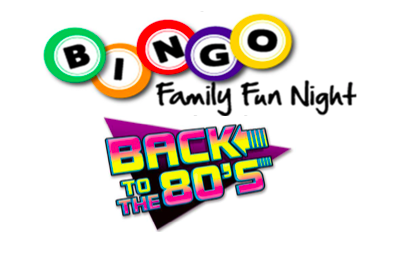# "Great Scott!"Bingo Night is TONIGHT!

## Hope to see everyone in theirTotally Rad 80's Attire!

If you pre-registered with a paper form or online, please stop by the Registration Tables to pick up your Bingo Cards, Pizza Vouchers, and Drink Tickets!

NOTE: We are still taking Walk Up Registrations (Cash/Credit Cards Accepted), but we only ordered 15 extra pizzas and they will be sold on a "first-come / first-serve" basis - so just a heads up that you might need to make your own plans for dinner.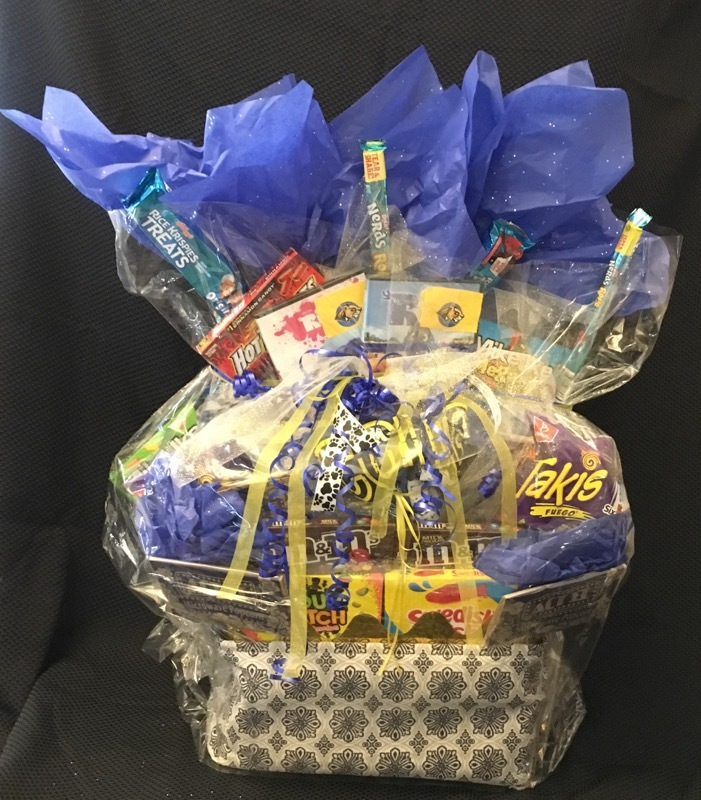# ALSO - Remember to bring CASH for the 50/50 Raffle and for our AWESOME Raffle Baskets.

## Bingo Daubers, Snacks & Drinkswill be on sale for \$1 each (CASH ONLY)

 table div table+table+table+table+table+table+table+table+table+table div table{width:100%;padding:0}table div table+table+table+table+table+table+table+table+table+table div table img{width:96.23%;padding:0;float:none}table div table+table+table+table+table+table+table+table+table+table div table td{width:100%;padding:0 1.88% 18px}/* styles */## REMINDER:SMHS Matadors in Training Clinic isSATURDAY (9/7)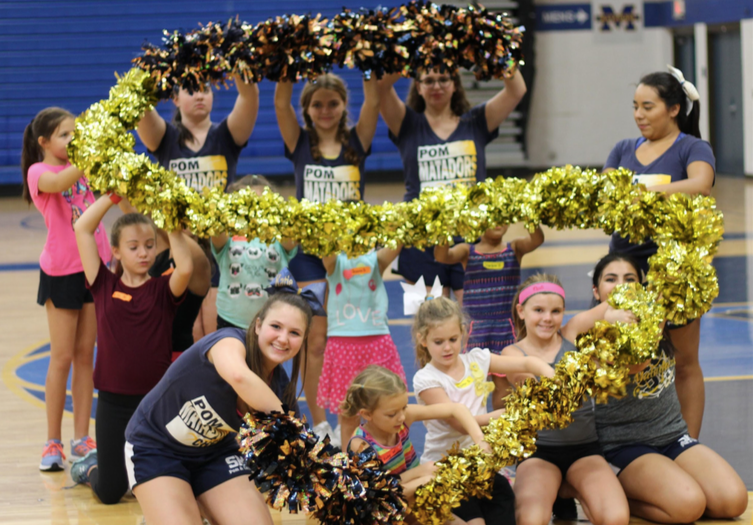# The Shadow Mountain High School Pom & Cheer Team is hosting their bi-annual Matadors In Training (MIT) clinic on Saturday, 9/7.

 /* styles */ Don't worry if your student can't attend this one, they do another one during Basketball season!
 table div table+table+table+table+table+table+table+table+table+table+table+table+table+table+table div table{width:100%;padding:0}table div table+table+table+table+table+table+table+table+table+table+table+table+table+table+table div table img{width:96.23%;padding:0;float:none}table div table+table+table+table+table+table+table+table+table+table+table+table+table+table+table div table td{width:100%;padding:0 1.88% 18px}/* styles */# Help our AMAZING Nurse Stephanie with completing the Health Screenings for our 500+ Students!## All help is greatly appreciated!

 table div table+table+table+table+table+table+table+table+table+table+table+table+table+table+table+table+table+table+table+table+table div table{width:100%;padding:0}table div table+table+table+table+table+table+table+table+table+table+table+table+table+table+table+table+table+table+table+table+table div table img{width:96.23%;padding:0;float:none}table div table+table+table+table+table+table+table+table+table+table+table+table+table+table+table+table+table+table+table+table+table div table td{width:100%;padding:0 1.88% 18px}/* styles */## Our next DC SPIRIT NIGHT is at HABIT BURGER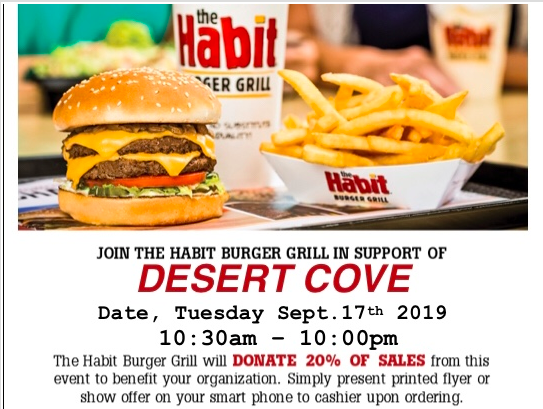table div table+table+table+table+table+table+table+table+table+table+table+table+table+table+table+table+table+table+table+table+table+table+table+table+table div table{width:100%;padding:0}table div table+table+table+table+table+table+table+table+table+table+table+table+table+table+table+table+table+table+table+table+table+table+table+table+table div table img{width:96.23%;padding:0;float:none}table div table+table+table+table+table+table+table+table+table+table+table+table+table+table+table+table+table+table+table+table+table+table+table+table+table div table td{width:100%;padding:0 1.88% 18px}/* styles */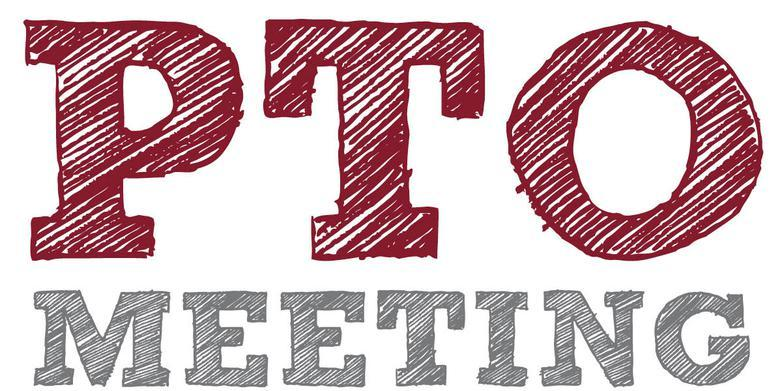# FREE Pizza & Childcare provided!

Can't make the meeting? No worries, Meeting Minutes are posted on our website:

www.desertcovepto.org/meetings

 table div table+table+table+table+table+table+table+table+table+table+table+table+table+table+table+table+table+table+table+table+table+table+table+table+table+table+table+table div table{width:100%;padding:0}table div table+table+table+table+table+table+table+table+table+table+table+table+table+table+table+table+table+table+table+table+table+table+table+table+table+table+table+table div table img{width:96.23%;padding:0;float:none}table div table+table+table+table+table+table+table+table+table+table+table+table+table+table+table+table+table+table+table+table+table+table+table+table+table+table+table+table div table td{width:100%;padding:0 1.88% 18px}/* styles */# VOLUNTEERS NEEDED EVERY DAY - 1 HOUR SHIFTS!!

 table.module-30{width:64.15%;padding:0}table div table+table+table+table+table+table+table+table+table+table+table+table+table+table+table+table+table+table+table+table+table+table+table+table+table+table+table+table+table+table+table div table{width:64.15%;float:none;margin-left:auto;margin-right:auto;padding:0}table div table+table+table+table+table+table+table+table+table+table+table+table+table+table+table+table+table+table+table+table+table+table+table+table+table+table+table+table+table+table+table div table a{border:0 none;text-decoration:none}table div table+table+table+table+table+table+table+table+table+table+table+table+table+table+table+table+table+table+table+table+table+table+table+table+table+table+table+table+table+table+table div table img{width:100%!important;border:0 none;text-decoration:none}table div table+table+table+table+table+table+table+table+table+table+table+table+table+table+table+table+table+table+table+table+table+table+table+table+table+table+table+table+table+table+table div table td{width:100%;padding:0}/* styles */

You can Shop ONLINE before & after the dates of the Fair at the School!

## And be sure to check out the new eWallet feature! No more losing cash that was sent to school with your student!

 table div table+table+table+table+table+table+table+table+table+table+table+table+table+table+table+table+table+table+table+table+table+table+table+table+table+table+table+table+table+table+table+table+table+table+table div table{width:100%;padding:0}table div table+table+table+table+table+table+table+table+table+table+table+table+table+table+table+table+table+table+table+table+table+table+table+table+table+table+table+table+table+table+table+table+table+table+table div table img{width:96.23%;padding:0;float:none}table div table+table+table+table+table+table+table+table+table+table+table+table+table+table+table+table+table+table+table+table+table+table+table+table+table+table+table+table+table+table+table+table+table+table+table div table td{width:100%;padding:0 1.88% 18px}/* styles */table.module-35{width:99.06%;padding:0}table div table+table+table+table+table+table+table+table+table+table+table+table+table+table+table+table+table+table+table+table+table+table+table+table+table+table+table+table+table+table+table+table+table+table+table+table div table{width:99.06%;float:none;margin-left:auto;margin-right:auto;padding:0}table div table+table+table+table+table+table+table+table+table+table+table+table+table+table+table+table+table+table+table+table+table+table+table+table+table+table+table+table+table+table+table+table+table+table+table+table div table a{border:0 none;text-decoration:none}table div table+table+table+table+table+table+table+table+table+table+table+table+table+table+table+table+table+table+table+table+table+table+table+table+table+table+table+table+table+table+table+table+table+table+table+table div table img{width:100%!important;border:0 none;text-decoration:none}table div table+table+table+table+table+table+table+table+table+table+table+table+table+table+table+table+table+table+table+table+table+table+table+table+table+table+table+table+table+table+table+table+table+table+table+table div table td{width:100%;padding:0}/* styles */

# There are SO many ways to stay connected this year! Choose one or more that work best for your family!

## DC PTO Remind App (TEXT messages)

 table div table+table+table+table+table+table+table+table+table+table+table+table+table+table+table+table+table+table+table+table+table+table+table+table+table+table+table+table+table+table+table+table+table+table+table+table+table+table+table+table div table{width:100%;padding:0}table div table+table+table+table+table+table+table+table+table+table+table+table+table+table+table+table+table+table+table+table+table+table+table+table+table+table+table+table+table+table+table+table+table+table+table+table+table+table+table+table div table img{width:96.23%;padding:0;float:none}table div table+table+table+table+table+table+table+table+table+table+table+table+table+table+table+table+table+table+table+table+table+table+table+table+table+table+table+table+table+table+table+table+table+table+table+table+table+table+table+table div table td{width:100%;padding:0 1.88% 18px}/* styles */table div table+table+table+table+table+table+table+table+table+table+table+table+table+table+table+table+table+table+table+table+table+table+table+table+table+table+table+table+table+table+table+table+table+table+table+table+table+table+table+table+table div table{width:100%;padding:0}table div table+table+table+table+table+table+table+table+table+table+table+table+table+table+table+table+table+table+table+table+table+table+table+table+table+table+table+table+table+table+table+table+table+table+table+table+table+table+table+table+table div table img{width:96.23%;padding:0;float:none}table div table+table+table+table+table+table+table+table+table+table+table+table+table+table+table+table+table+table+table+table+table+table+table+table+table+table+table+table+table+table+table+table+table+table+table+table+table+table+table+table+table div table td{width:100%;padding:0 1.88% 18px}/* styles */We have created a page on the PTO website where you can access the following communications:

▪ Desert Cove PTO eNews archives
▪ PV School District eNews
▪ United Parent Council Bulletins & Meeting Info
 ▪ Desert Cove PTO eNews archives
 ▪ PV School District eNews
 ▪ United Parent Council Bulletins & Meeting Info